Main Radar Home Radar theory Home AP3302 Pt3 Contents AP3302 Pt3 Section 1 Contents AP 3302 Pt. 3 Section 1 CHAPTER 5 Basic outline of CW radar Radar Altimeters Using Frequency Modulation The principles of frequency modulation are considered elsewhere in these notes (see pp 388 and 423 of AP 3302, Part lB). Basically in a f.m. transmitter the carrier frequency is caused to change at a rate determined by the frequency of the modulating signal and by an amount deter-mined by the amplitude of the modulating signal. The transmitter works continuously and produces a constant-amplitude c.w. output whose frequency is varied by the modulating signal.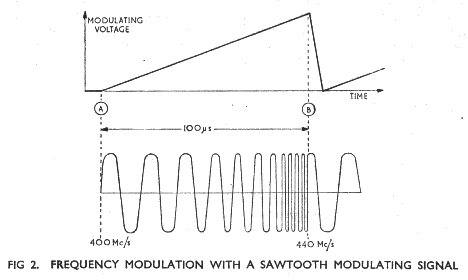Let us suppose that the frequency of a f.m. transmitter is caused to deviate at a constant rate by using a sawtooth waveform as the modulating signal (Fig 2). At point A the carrier frequency is, say, 400 Mc/s. At point B, 100 us later, the frequency is, say, 440 Mc/s. Since the change in frequency is linear we can say that the transmitter frequency is changing by 40 Mc/s every 100 us. Let us see how this principle is applied in the f.m.c.w. radar altimeter.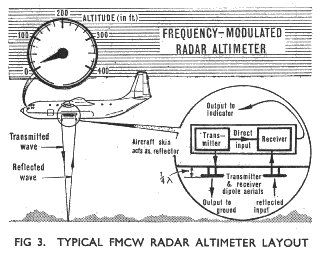Fig 3 illustrates the layout of a typical f.m.c.w. radar altimeter in an aircraft. Let us assume that the output frequency is changing as described above and that at a given instant of time it is 410 Mc/s. The wave of this frequency is radiated downwards and reflected from the ground to be picked up by the receiver aerial. The wave takes a definite time to travel over this path so that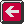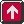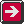Previous page To top of this page Next Page

Constructed by Dick Barrett
Email: